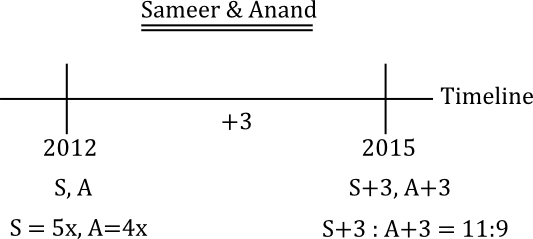## Target SBI PO 2017: Problems on Ages

WORD PROBLEMS BASED ON AGES
Problems on ages are one of the most frequently asked questions in any of the Competitive examinations and which are also among the easiest of the questions, you could attempt in the examinations. With the knowledge of solving linear equations, you can solve any problem related to ages. I will try to explain the general approach of solving questions related to problems based on ages.
General Approach for Problems based on Ages
Irrespective of what the question is, you would prepare what is called as TIMELINE. Once you draw the timeline, the whole problem becomes so easy to solve.
Let me explain with an example.Let’s say the question talks of a father and his son, whose present ages, we need to find out. So, you would consider their ages as F & S.
Step 1 :  First step is to draw a timeline as shown in the figure. As the present ages are not known, we represent their ages in 2012 (Current year) as F & S. There will be additional data given in the question, how the Father’s and Son’s ages are related.
Step 2 :  If in the question, you have something like 5 years ago, you go back 5 years from 2012, that is, 2007. So in 2007, Father’s age would be 5 years less, so (F-5). Similarly , Son’s age would be also 5 years less in which case, Son’s age in 2007 would be (S-5).
Step 3 :  Suppose the data concerning the Father’s and Son’s age is in the future, you will go so many years in the future. Let’s suppose 6 years hence, in which case the year would be 2018. The father’s age would then be (F+6) and son’s age would be (S+6).
Generally, you will have 2 linear equations with 2 unknowns. You can solve for the ages by solving linear equations.
Example 1: Four years ago Ram was four times as old as Shyam. Today Ram is twice as old. How old is Ram now?
Solution: Let’s prepare the timeline with the data given.Step 1 : Once you prepare the timeline, the 2 linear equations are very clear.  At present, Ram is twice as old as Shyam. So, R =2S
Step 2 : Four years ago, that means in 2008 ( Considering the present age as 2012). So, Ram’s age in 2008 was R-4 and Shyam’s age was S-4. It is given the question, that Ram’s age in 2008 was 4 times Shyam’s age in 2008. So, we have the second linear equation  R-4 = 4(S-4)
Solving the 2 linear equations, we have :
R – 4 = 4(S-4)
R –  4 =4(R/2) – 16
R – 4 = 2R =16
R =12 and S would be 6
Example 2: Present ages of Sameer and Anand are in the ratio of 5 : 4 respectively. Three years hence, the ratio of their ages will become 11 : 9 respectively. What is Anand’s present age in years?
Solution:  Let’s prepare the timeline with the data given.Step 1 : Once you prepare the timeline, the 2 linear equations are very clear. At present, Sameer and Anand’s ages are in the ratio 5:4
Let S=5x, A=4x
Step 2 : After 3 years, that is in 2015 ( Considering the present age as 2012). So, Sameer’s age in 2015 would be S+3 and Anand’s age would be A+3.
It is given in 2015, S+3:A+3 = 11:9
Solving the 2 linear equations, we have :
9( 5x + 3 ) = 11( 4x + 3)
45x + 27 = 44x + 33
x = 6
Sameer’s Present Age is 5x = 30
Anand’s Present Age is 4x = 24
Example 3: The sum of the ages of father & his son is 45 years.  Five years ago the product of their ages was 34. Find the ages of the son & the father now ?
Solution : Let’s prepare the timeline with the data given.Step 1 : Once you prepare the timeline, the 2 linear equations are very clear.  At present, father’s and son’s age together is 45. So F+S=45
Step 2 : Five years ago, that means in 2007 ( Considering the present age as 2012) Father’s age was (F-5) and son’s age was (S-5). It is given that product of their ages in 2007 was 34. So, the equation would be (F-5)(S-5)=34.
Now, we have 2 equations:
(F-5)(S-5)=34 & F+S =34
Notice the first equation is not linear. So, one approach would be Use the answers given as options & use the reverse techniques.
Alternatively, Solve the 2 equations:
(F-5)(45 – F – 5) = 34
(F-5)(40 – F) = 34
40F – F2 – 200 + 5F =34
-F2 + 45F – 234 = 0
F2 – 45F + 234 = 0
(F – 39)(F – 6 ) = 0
So F is either 39 or 6
If we take F=6, then F+S = 45 & S=39.
Son’s age cannot be greater than the father’s age. We can ignore that.
The present age of Father is 39 and that of son is 6 years.